# Ex 13.2 NCERT Solutions- Surface Areas and Volumes Class 9 Notes | EduRev

## Class 9 : Ex 13.2 NCERT Solutions- Surface Areas and Volumes Class 9 Notes | EduRev

The document Ex 13.2 NCERT Solutions- Surface Areas and Volumes Class 9 Notes | EduRev is a part of the Class 9 Course Mathematics (Maths) Class 9.
All you need of Class 9 at this link: Class 9

Question 1. The curved surface area of a right circular cylinder of height 14 cm is 88 cm2. Find the diameter of the base of the cylinder.
Solution:
Let ‘r ’ be the radius of the cylinder.
Here, height (h) = 14 cm and curved surface area = 88 cm2
∵ Curved surface area of a cylinder = 2πrh
∴ 2 πrh = 88
⇒ 2 x (22/7)r x 14 = 88

⇒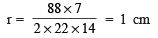⇒ Diameter = 2 x r = 2 x 1 cm = 2 cm

Question 2. It is required to make a closed cylindrical tank of height 1 m and base diameter 140 cm from a metal sheet. How many square metres of the sheet are required for the same?
Solution:
Here, height (h) = 1 m
∵ Diameter of the base = 140 cm = 1.40 m
∴ Radius (r) = (1.40/2) = 0.70 m
Total surface area of the cylinder = 2πr (h + r)
= 2 x(22/7)x 0.70 (1 + 0.70) m
= 2 x 22 x 0.10 (1.70) m2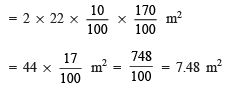Hence, the required sheet is 7.48 m

Question 3. A metal pipe is 77 cm long. The inner diameter of a cross section is 4 cm, the outer diameter being 4.4 cm (see figure) Find its. (i) inner curved surface area, (ii) outer curved surface area, (iii) total surface area.
Solution:
Length of the metal pipe = 77 cm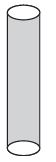∵ It is in the form of a cylinder.
∴ Height (h) of the cylinder = 77 cm Inner diameter = 4 cm
⇒ Inner radius (r) =(4/2)= 2 cm
Outer diameter = 4.4 cm
⇒ Outer radius (R) = (4.4/2)= 2.2 cm

(i)  ∵ Curved surface area = 2πrh = 2 x (22/7) x 2 x 77 cm2

= 2 x 22 x 2 11 cm2 = 968 cm2

(ii)  Outer curved surface area = 2πrh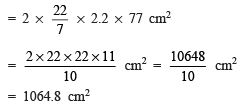(iii) Total surface area

= [Inner curved surface] + [outer curved surface area] + [Two base circular lamina]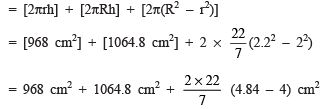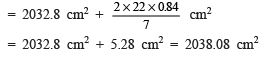Question 4. The diameter of a roller is 84 cm and its length is 120 cm. It takes 500 complete revolutions to move once over to level a playground. Find the area of the playground in m2.
Solution:
The roller is in the form of a cylinder diameter of the roller = 84 cm
⇒ Radius of the cylinder =(84/2)
= 42 cm
Length of the roller = 120 cm
⇒ Height of the cylinder (h) = 120 cm
∴ Curved surface area of the roller (cylinder) = 2 πrh
= 2 x (22/7)x 42 x 120 cm
= 2 x 22 x 6 x 120 cm= 31680 cm2
∴ Area of the playground levelled in one revolution of the roller = 31680 cm2

=   (31680 / 10000) m2

⇒ Area levelled in 500 revolutions = 500 x (31680 / 10000) m2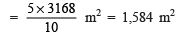Question 5. A cylindrical pillar is 50 cm in diameter and 3.5 m in height. Find the cost of painting the curved surface of the pillar at the rate of ₹ 12.50 per m2.
Solution:
Diameter of the pillar = 50 cm
⇒ Radius (r) = (50/2) = 25 cm = (1/4)m
Height (h) = 3.5 m = 3.50 m
∵ Curved surface area of a cylinder = 2πrh
∴ Curved surface area of a cylinder = 2 x (22/7) x(1/4)x 3.50 m2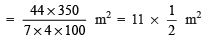⇒ Curved surface area to be painted = (11/2)m2
Cost of painting ∵ Rate of painting = ₹ 1250 per m2

∴ Cost of Painting 11/2 m2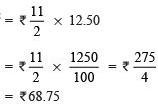Question 6. Curved surface area of a right circular cylinder is 4.4 m2. If the radius of the base of the cylinder is 0.7 m, find its height.
Solution:
Radius (r) = 0.7 m Let height of the cylinder be ‘h’ metres.
∴ Curved surface area = 2πrh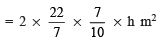But the curved surface area is 4.4 m2.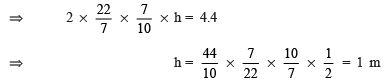Thus, the required height is 1 metre.

Question 7. The inner diameter of a circular well is 3.5 m. It is 10 m deep. Find: (i) its inner curved surface area. (ii) the cost of plastering this curved surface at the rate of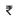40 per m2.
Solution:
Inner diameter of the well = 3.5 m
⇒ Radius of the well (cylinder) (r) =(3.5/2)
.
Depth of the well (= height of the cylinder) h = 10 m
∴ (i) Inner curved surface area = 2πrh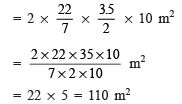(ii) Cost of plastering
∵ Rate of plastering = ₹40 per m2
∴ Total cost of plastering 110 m2 = ₹110 x 40 = ₹4400

Question 8. In a hot water heating system, there is a cylindrical pipe of length 28 m and diameter 5 cm. Find the total radiating surface in the system.
Solution:
Length of the cylindrical pipe = 28 m
⇒ h = 28 m
Diameter of the pipe = 5 cm
∴ Radius (r) = (5/2) cm = (5/200) m

∴ Curved surface area = 2πrh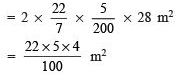= (440/100)m2 = 4.40 m2

Thus, the total radiating surface is 4.40 m2

Question 9. Find: (i) the lateral or curved surface area of a closed cylindrical petrol storage tank that is 4.2 m in diameter and 4.5 m high.
(ii) how much steel was actually used if (1/12) of the steel actually used was wasted in making the tank.
Solution:
The storage tank is in the form of a cylinder, and diameter of the tank = 4.2 m
∴ The radius (r) = (42/2) = 2.1 m
Height (h) = 4.5 m

Now, (i) Lateral (or curved) surface area = 2πrh
= 2 x (22/7) x 2.1 x 4.5 m2
= 2 x 22 x 0.3 x 4.5 m2
= 59.4 m2

(ii) Total surface area of the tank = 2πr(r + h)
= 2 x (22/7) x 2.1 (2.1 + 4.5) m2
= 44 x 0.3 x 6.6 m= 87.12 m

Let actual area of the steel used = x m2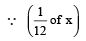steel was wasted.

∴ Area of steel that wasted = (1/12) x x
=  (x/12)m2

⇒ Area of steel used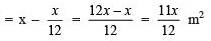⇒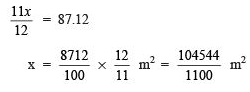Thus, the required area of the steel that was actually used is 95.04 m2

Question 10. In the figure you see the frame of a lampshade. It is to be covered with a decorative cloth. The frame has base diameter of 20 cm and height of 30 cm. A margin of 2.5 cm is to be given for folding it over the top and bottom of the frame. Find how much cloth is required for covering the lampshade.
Solution:
The lampshade is in the form of a cylinder, where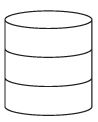r= (20/2)= 10 cm Height = 30 cm.
∵ A margin of 2.5 cm is to be added to top and bottom.
∴ Total height of the cylinder h = 30 cm + 2.5 cm + 2.5 cm = 35 cm
Now, curved surface area = 2 πrh = 2 x (22/7)x 10 x (35) cm
= 2 x 22 x 10 x 5 cm
= 2200 cm2
Thus, the required area of the cloth = 2200 cm2

Question 11. The students of a Vidyalaya were asked to participate in a competition for making and decorating penholders in the shape of a cylinder with a base, using cardboard. Each penholder was to be of radius 3 cm and height 10.5 cm. The Vidyalaya was to supply the competitors with cardboard. If there were 35 competitors, how much cardboard was required to be bought for the competition?
Solution:
Here, the penholders are in the form of cylinders Radius of a cylinder (r) = 3 cm
Height of a cylinder (h) = 10.5 cm
Since, a penholder must be open from the top.
∴ Surface area of a penholder (cylinder) = [Lateral surface area] + [Base area]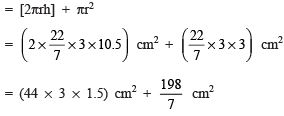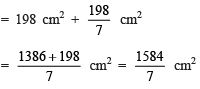⇒ Surface area of 35 penholders =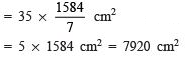[∵ There are 35 competitors]

Thus, 7920 cm2 of cardboard was required to be bought.

SURFACE AREA OF A RIGHT CIRCULAR CONE

If we rotate a right angled triangular lamina AOB about OA, a cone is generated, as shown in the adjoining figure. OA is its height (h) and OB is a radius of its base (r) such that OA ⊥ OB. Its base is a circle with centre O. The length AB = l is called its slant height.
We have: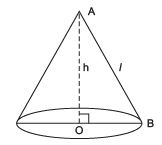(i) l (slant height) =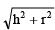(ii) Curved (= Lateral surface) area = πrl
(iii) Total surface area = Lateral surface area + Base area = πrl + πr2 = πr(l + r)

Note: In the following πroblems assume π =  (22/7)unless stated otherwise.

Offer running on EduRev: Apply code STAYHOME200 to get INR 200 off on our premium plan EduRev Infinity!

## Mathematics (Maths) Class 9

190 videos|233 docs|82 tests

,

,

,

,

,

,

,

,

,

,

,

,

,

,

,

,

,

,

,

,

,

;